# AEPA Math: Limits Chapter Exam

Exam Instructions:

Choose your answers to the questions and click 'Next' to see the next set of questions. You can skip questions if you would like and come back to them later with the yellow "Go To First Skipped Question" button. When you have completed the practice exam, a green submit button will appear. Click it to see your results. Good luck!

### Page 1

#### Question 1 1. The ceiling function is graphically translated as a stepwise function. What is the limit of f(x) = ceil(x) as x approaches 4.5?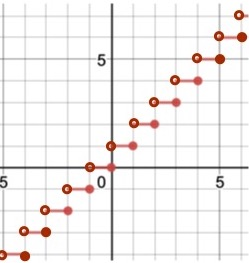#### Question 2 2. The floor function is graphically represented as a stepwise function. What is the limit of f(x) = floor(x) as x approaches 4?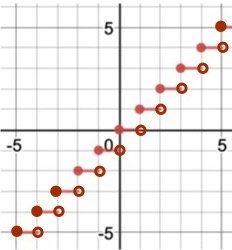#### Question 3 3. Which of the following is true?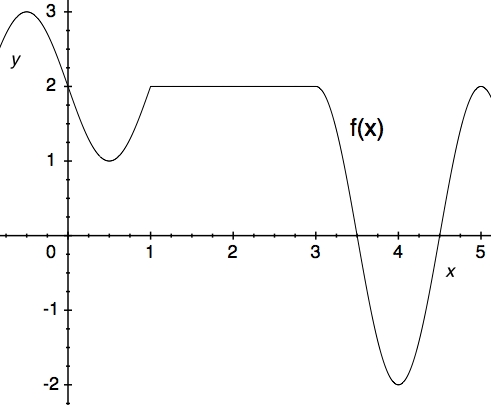#### Question 4 4. Using the graph, solve z+1.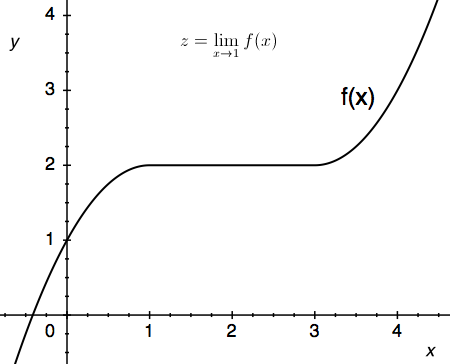#### Question 5 5. Use the given formula to solve for z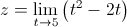### Page 2

#### Question 6 6. Use the given graph and formula to solve for z.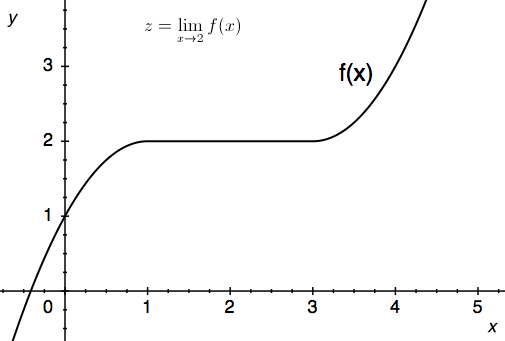#### Question 7 7. Using the given data, solve for y.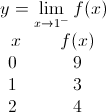#### Question 8 8. Use the given graph and formula to solve for z.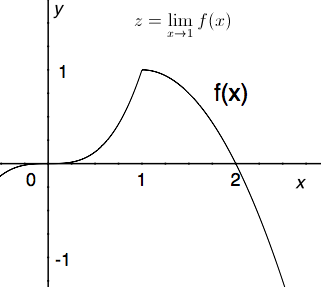#### Question 9 9. Use the graph to solve for z.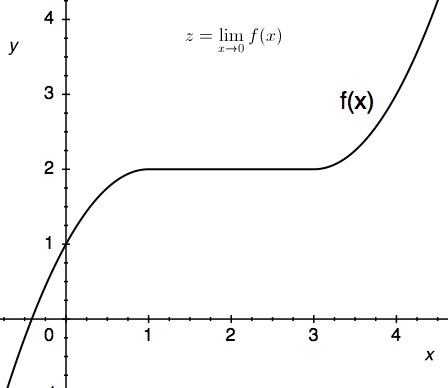### Page 3

#### Question 11 11. Use the graph to solve for z.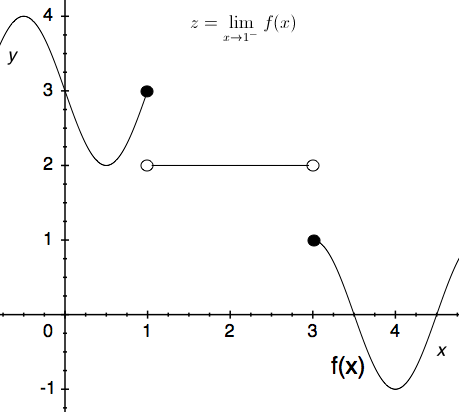#### Question 12 12. Using the given data, solve for z.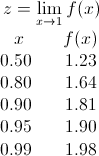#### Question 13 13. Use the graph to solve for z.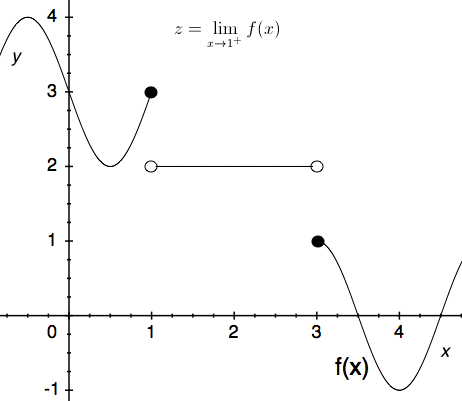### Page 4

#### Question 18 18. Use the graph to solve for z.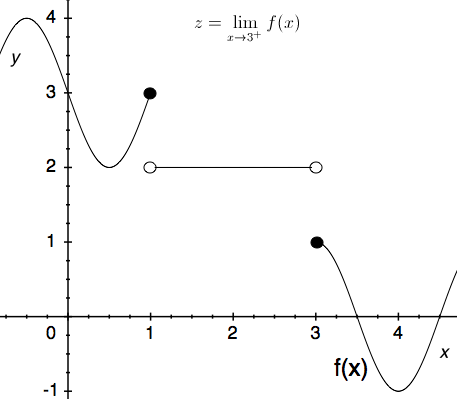#### Question 19 19. Using the given data, solve for y.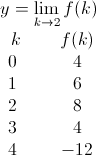#### AEPA Math: Limits Chapter Exam Instructions

Choose your answers to the questions and click 'Next' to see the next set of questions. You can skip questions if you would like and come back to them later with the yellow "Go To First Skipped Question" button. When you have completed the practice exam, a green submit button will appear. Click it to see your results. Good luck!

Support